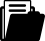Meine Position
i
Ein Tipp vom Eldar Team
Je mehr Sie bestellen, desto grösser Ihr RabattBuch
Buch
Fachbuch
1996

# Integral Transformations, Operational Calculus, and Generalized Functions

ISBN
EAN
978-0-7923-4183-3
9780792341833
Artikel-Nr.
EN4LNZ9
Kostenloser Versand
CHF
134.78
Anzahl
1
Maximale
Lieferzeit
13
Arbeitstage
Freitag
15.10.2021Beschreibung
It is not the object of the author to present comprehensive cov erage of any particular integral transformation or of any particular development of generalized functions, for there are books available in which this is done. Rather, this consists more of an introductory survey in which various ideas are explored. The Laplace transforma tion is taken as the model type of an integral transformation and a number of its properties are developed; later, the Fourier transfor mation is introduced. The operational calculus of Mikusinski is pre sented as a method of introducing generalized functions associated with the Laplace transformation. The construction is analogous to the construction of the rational numbers from the integers. Further on, generalized functions associated with the problem of extension of the Fourier transformation are introduced. This construction is anal ogous to the construction of the reals from the rationals by means of Cauchy sequences. A chapter with sections on a variety of trans formations is adjoined. Necessary levels of sophistication start low in the first chapter, but they grow considerably in some sections of later chapters. Background needs are stated at the beginnings of each chapter. Many theorems are given without proofs, which seems appro priate for the goals in mind. A selection of references is included. Without showing many of the details of rigor it is hoped that a strong indication is given that a firm mathematical foundation does actu ally exist for such entities as the "Dirac delta-function".Stichwörter
Fourier transform
calculus
differential equation
distribution
integral transform
operational calculus
Zielgruppe
Research
Inhaltsverzeichnis
Preface. 1. Laplace Transformations. 2. Mikusinski Operators. 3. Fourier Transformations. 4. Generalized Functions. 5. Other Transformations. References. Appendices: A. The Titchmarsh Theorem. B. Inversion Integrals. C. Interchange of Order of Infinite Processes. D. Definitions and Properties of Some Special Functions. Tables of Transforms: 1. Laplace. 2. Inverse Laplace. 3. Fourier. 4. Fourier Cosine. 5. Fourier Sine. 6. Mellin. 7. Power Series. 8. Finite Fourier. 9. Finite Fourier Cosine. 10. Finite Fourier Sine. 11. Finite Laplace. 12. Two-Dimensional Laplace. 13. Inverse Two-Dimensional Laplace. Index.Herausgeber/-in
Publikation
Vereinigte Staaten
31.07.1996Sprache
EnglischFormat
Hardcover
240 SeitenWeitere FormateVersand
Kostenloser Versand: Schweiz & Liechtenstein
Für den Versand nach Deutschland oder Frankreich werden die Versandgebühren der Schweizerischen Post berechnet. Diese werden Ihnen im Warenkorb für Ihre gesamte Bestellung berechnet.Themen
Unterkategorie
Analysis
Zurück
Zum Start